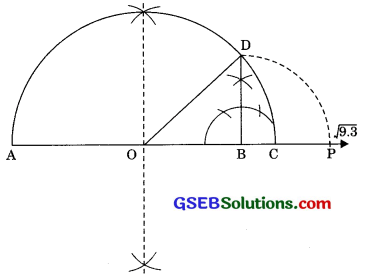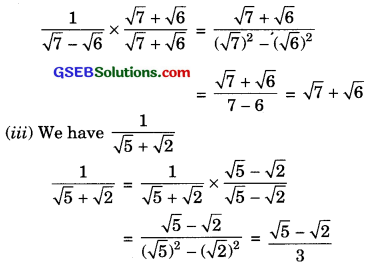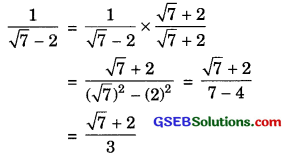# GSEB Solutions Class 9 Maths Chapter 1 Number Systems Ex 1.5

Gujarat Board GSEB Solutions Class 10 Maths Chapter 1 Number Systems Ex 1.5 Textbook Questions and Answers.

## Gujarat Board Textbook Solutions Class 9 Maths Chapter 1 Number Systems Ex 1.5

Question 1.
Classify the following numbers as rational or irrational.
Solution:
(i) 2 – $$\sqrt{5}$$
(ii) (3 + $$\sqrt{23}$$) – $$\sqrt{23}$$
(iii) $$\frac{2 \sqrt{7}}{7 \sqrt{7}}$$
(iv) $$\frac{1}{\sqrt{2}}$$
(v) 2π
Solution:
(i) 2 is a rational number and $$\sqrt{5}$$ is an irrational number, then 2 – $$\sqrt{5}$$ is an irrational number.
(The difference of rational number and irrational number is an irrational number)(ii) (3 + $$\sqrt{23}$$) – $$\sqrt{23}$$ = 3 + $$\sqrt{23}$$ – $$\sqrt{23}$$ = 3 (3 is a rational number)

(iii) $$\frac{2 \sqrt{7}}{7 \sqrt{7}}$$ = $$\frac { 2 }{ 7 }$$ = which is a rational number.

(iv) $$\frac{1}{\sqrt{2}}$$
Here, 1 is rational number and $$\sqrt{2}$$ is an irrational number.
∴ The quotient of a non-zero rational number with an irrational number we get an irrational number.
∴ $$\frac{1}{\sqrt{2}}$$ is an irrational number.

(v) 2π
Here, 2 is a rational number (2 ≠ 0)
and π is an irrational number.
∴ π is an irrational number.
(Because the product of a non-zero rational number with an irrational number is an irrational number).Question 2.
Simplify each of the following expressions:
(i) (3 + $$\sqrt{3}$$) (2 + $$\sqrt{2}$$)
(ii) (3 + $$\sqrt{3}$$) (3 – $$\sqrt{3}$$)
(iii) ($$\sqrt{5}$$ + $$\sqrt{2}$$)2
(iv) ( $$\sqrt{5}$$ – $$\sqrt{2}$$) ($$\sqrt{5}$$ + $$\sqrt{2}$$)
Solution:
(i) (3 + $$\sqrt{3}$$) (2 + $$\sqrt{2}$$)
= 3(2 + $$\sqrt{2}$$) + $$\sqrt{3}$$(2 + $$\sqrt{2}$$)
= 6 + 3$$\sqrt{2}$$ + 2$$\sqrt{3}$$ + $$\sqrt{3}$$ x $$\sqrt{2}$$
= 6 + 3$$\sqrt{2}$$ + 2$$\sqrt{3}$$ + $$\sqrt{6}$$ (-$$\sqrt{a}$$ x $$\sqrt{b}$$ = $$\sqrt{ab}$$)

(ii) (3 + $$\sqrt{3}$$) (3 – $$\sqrt{3}$$)
= 32 – ($$\sqrt{3}$$)2
[(a – $$\sqrt{a}$$) (a + $$\sqrt{b}$$) = a2 – b]
= 9 – 3 = 6

(iii) ($$\sqrt{5}$$ + $$\sqrt{2}$$)2
= ( $$\sqrt{5}$$)2 + 2 $$\sqrt{5}$$ x $$\sqrt{2}$$ + ( $$\sqrt{2}$$)2
= 5 + 2$$\sqrt{10}$$ + 2 ( $$\sqrt{a}$$ x $$\sqrt{b}$$ = $$\sqrt{ab}$$)
= 7 + 27$$\sqrt{10}$$

(iv) ($$\sqrt{5}$$ – $$\sqrt{2}$$) ( $$\sqrt{5}$$ + $$\sqrt{2}$$)
= ($$\sqrt{5}$$)2 – ($$\sqrt{2}$$)2 = 5 – 2 = 3

Question 3.
Recall, n is defined as the ratio of the circumference (say c) of a circle to its diameter (say d). That is, π = $$\frac { c }{ d }$$. This seems to contradict the fact that n is irrational. How will you resolve this contradiction?
Solution:
π = $$\frac { c }{ d }$$
There is no contradiction as either c or d is irrational and hence n is an irrational.Question 4.
Represent $$\sqrt{9.3}$$ on the number line.
Solution:
We find $$\sqrt{9.3}$$ geometrically.
Let x = 79.3
1. Draw a number line and mark point A on it and take a distance AB = 9.3 units.
2. From point B mark a distance of 1 unit and mark the new point C.
3. Draw perpendicular bisector of AC and mark that point as O.
4. Draw a semicircle with centre O and radius OC.
5. Draw perpendicular at the point B which intersect the semicircle at point D then BD = $$\sqrt{9.3}$$.
6. Now from B as centre and radius equal to BD, draw an arc which intersects the number line at P. Thus, BP = $$\sqrt{9.3}$$units.AOBD is a right angled triangle. Also the
radius of circle r = $$\frac { AC }{ 2 }$$ i.e., $$\frac { x+1 }{ 2 }$$ units.
∴ OC = OD = OA = $$\frac { x+1 }{ 2 }$$ units
Now OB = OD = OA = $$\frac { x+1 }{ 2 }$$ units
⇒ OB = x – [ $$\frac { x+1 }{ 2 }$$ ]
⇒ OB = x – [ $$\frac { x+1 }{ 2 }$$ ]
By Pythagoras theorem, we have
BD2 = OD2 – OB2
[ $$\frac { x+1 }{ 2 }$$ ]2 – [ $$\frac { x-1 }{ 2 }$$ ]2 = $$\frac { 4x }{ 4 }$$ = x
∴ BD2 = x
⇒ BD = $$\sqrt{x}$$
Hence BD = $$\sqrt{9.3}$$
⇒ BD = 3.049Question 5.
Rationalise the denominators of the following:
(i) $$\frac{1}{\sqrt{7}}$$
(ii) $$\frac{1}{\sqrt{7 – 6}}$$
(iii) $$\frac{1}{\sqrt{7}-\sqrt{6}}$$
(iii) $$\frac{1}{\sqrt{5}+\sqrt{2}}$$
(iv) $$\frac{1}{\sqrt{7}-2}$$
Solution:
(i) We have $$\frac{1}{\sqrt{7}}$$
Multiplying by $$\sqrt{7}$$ to numerator and denominator, we get
$$\frac{1}{\sqrt{7}}$$ x $$\frac{\sqrt{7}}{\sqrt{7}}$$ x $$\frac{\sqrt{7}}{7}$$

(ii) $$\frac{1}{\sqrt{7 – 6}}$$
Multiplying the numerator and denominator by $$\sqrt{7}$$ + $$\sqrt{7}$$, we get(iv) $$\frac{1}{\sqrt{7}-2}$$
Multiplying the numerator and denominator by $$\sqrt{7}$$ + 2, we get